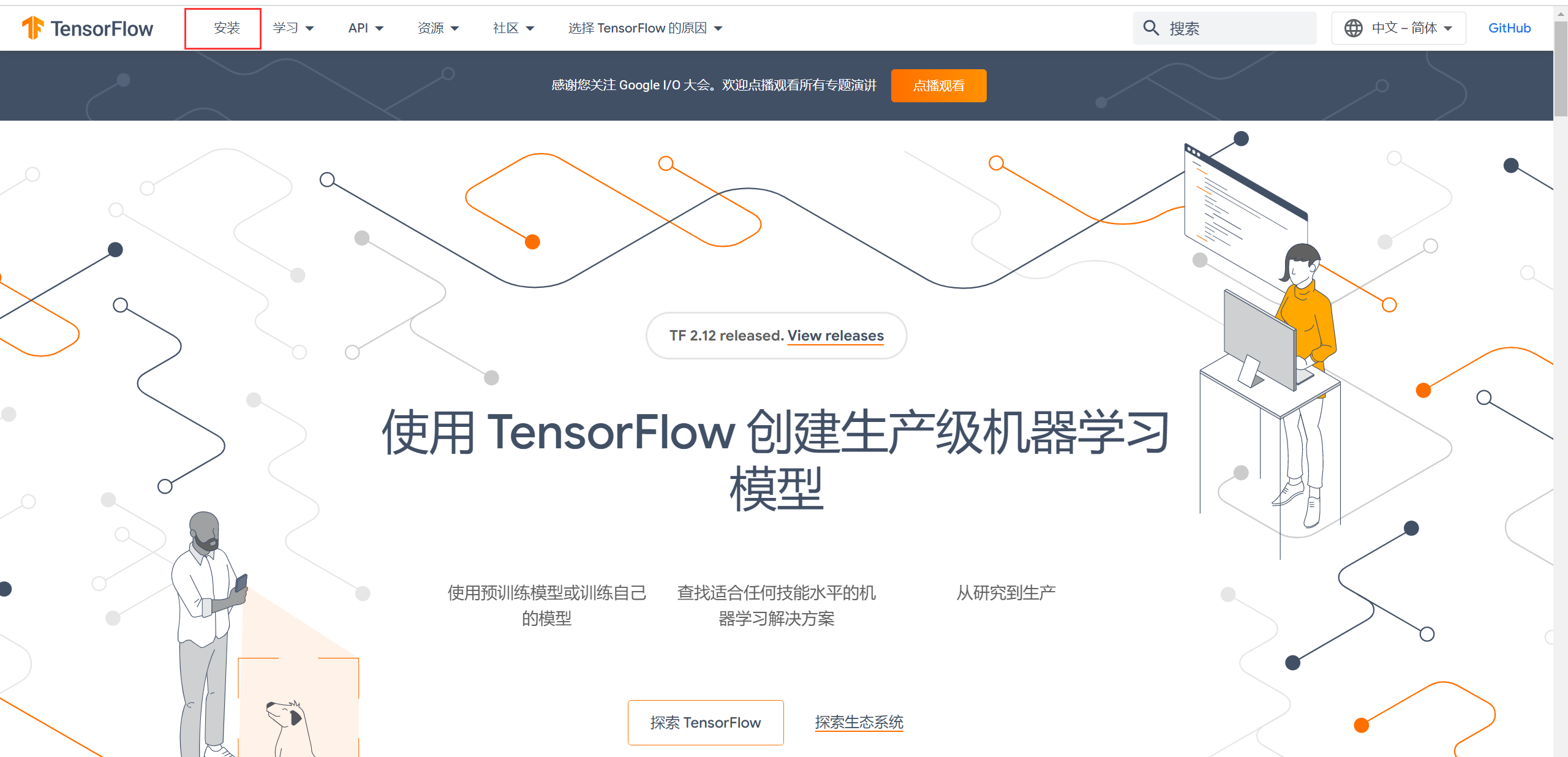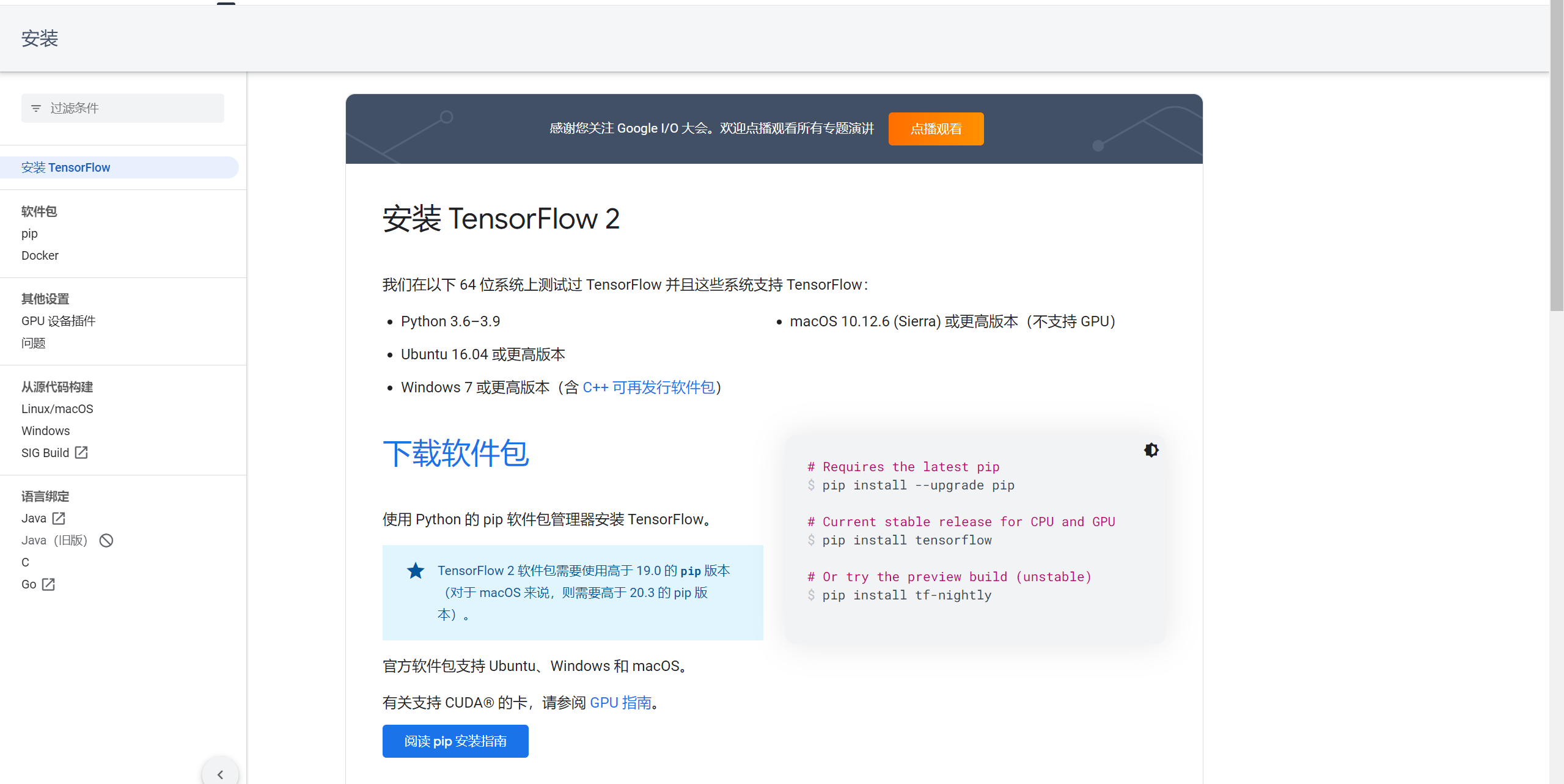# 安装 TensorFlow

TensorFlow 是什么？它是一个开源的机器学习框架，由 Google Brain 团队开发。它支持各种各样的机器学习算法和技术，包括深度学习、强化学习、自然语言处理等，并提供了一种易于使用的 API，使得开发者可以方便地构建和训练模型。TensorFlow 还支持分布式训练，可以通过多个计算机节点并行处理数据，提高训练效率。在开始 TensorFlow 编程之前，需要先安装 TensorFlow。推荐使用 pip 进行安装。在命令行中输入以下命令即可安装最新版本的 TensorFlow：

`pip install tensorflow`

# TensorFlow 基础概念

```import tensorflow as tf
​
# 创建常量节点
a = tf.constant(2)
b = tf.constant(3)
​
# 创建加法节点
​
# 创建 TensorFlow 会话
sess = tf.Session()
​
# 运行计算图，并输出结果
result = sess.run(c)
print(result)
​
# 关闭 TensorFlow 会话
sess.close()```

# TensorFlow 常用 API

```import tensorflow as tf
​
# 创建常量节点
a = tf.constant(2)
b = tf.constant(3)
​
# 创建变量节点
x = tf.Variable(0, name='x')
​
# 创建加法节点
​
# 创建赋值节点
assign_op = tf.assign(x, c)
​
# 创建 TensorFlow 会话
sess = tf.Session()
​
# 初始化变量
sess.run(tf.global_variables_initializer())
​
# 运行计算图，并输出结果
result = sess.run(assign_op)
print(result)
print(sess.run(x))
​
# 关闭 TensorFlow 会话
sess.close()```

```import tensorflow as tf
​
# 创建占位符节点
x = tf.placeholder(tf.float32, [None, 2])
y = tf.placeholder(tf.float32, [None, 1])
​
# 创建变量节点
w = tf.Variable(tf.zeros([2, 1]))
b = tf.Variable(tf.zeros())
​
# 创建线性模型
y_pred = tf.matmul(x, w) + b
​
# 创建损失函数节点
loss = tf.reduce_mean(tf.square(y_pred - y))
​
# 创建训练操作节点
​
# 生成数据集
import numpy as np
x_train = np.random.rand(100, 2)
y_train = np.sum(x_train, axis=1, keepdims=True)
​
# 创建 TensorFlow 会话
sess = tf.Session()
​
# 初始化变量
sess.run(tf.global_variables_initializer())
​
# 训练模型
for i in range(1000):
_, loss_val = sess.run([train_op, loss], feed_dict={x: x_train, y: y_train})
if i % 100 == 0:
print('step {}: loss={}'.format(i, loss_val))
​
# 关闭 TensorFlow 会话
sess.close()```

Keras API Keras 是一个高阶的深度学习 API，可以为 TensorFlow 提供易于使用的抽象层，使得开发者可以更加方便地构建和训练深度学习模型。Keras 实现了几乎所有常见的深度学习算法和技术，例如卷积神经网络、循环神经网络、自动编码器等。

```import tensorflow as tf
from tensorflow import keras
​
# 创建数据集
(x_train, y_train), (x_test, y_test) = keras.datasets.mnist.load_data()
​
# 构建模型
model = keras.Sequential([
keras.layers.Flatten(input_shape=(28, 28)),
keras.layers.Dense(128, activation=tf.nn.relu),
keras.layers.Dense(10, activation=tf.nn.softmax)
])
​
# 编译模型
loss='sparse_categorical_crossentropy',
metrics=['accuracy'])
​
# 训练模型
model.fit(x_train, y_train, epochs=5)
​
# 评估模型
test_loss, test_acc = model.evaluate(x_test, y_test)
print('Test accuracy:', test_acc)```

# 结论

TensorFlow 提供了丰富的 API 和工具，使得开发者可以方便地构建和训练机器学习模型。本教程介绍了 TensorFlow 的基础概念和常用 API，包括常量和变量、占位符和 Keras API 等。这些知识是使用 TensorFlow 进行机器学习和深度学习研究的必备基础。

...全文
46 回复 打赏 收藏 转发到动态 举报DeepLearning学习笔记以及Tensorflow、Pytorch的使用心得笔记
DeepLearning学习笔记以及Tensorflow、Pytorch的使用心得笔记。Dr. Sure会不定时往项目中添加他看到的最新的技术，欢迎批评指正。Tensorflow Objection_Detection API 使用心得
Tensorflow Objection_Detection API 使用心得Tensorflow安装Anaconda的安装玩API碰到大坑Objection Detection API 总结 最近这个星期根据网上的一些TF教程，开始学着自己做hand_detection玩一下，但是捣鼓来捣鼓去。总是在TF安装包、Anaconda安装等问题上一直来回浪费时间。这篇文章主要是说一下博主玩这个API遇...Tensorflow 中Tfrecords的使用心得TensorFlow源代码调试与修改TensorFlow入门学习教程

1,278发帖与我相关我的任务
android 企业社区• 近7日
• 近30日
• 至今